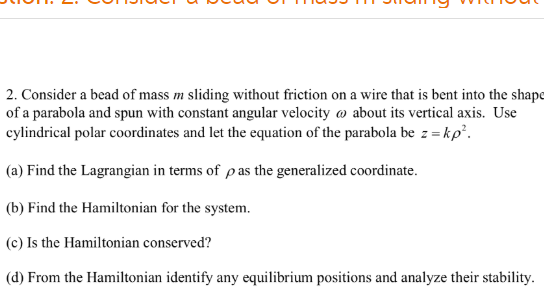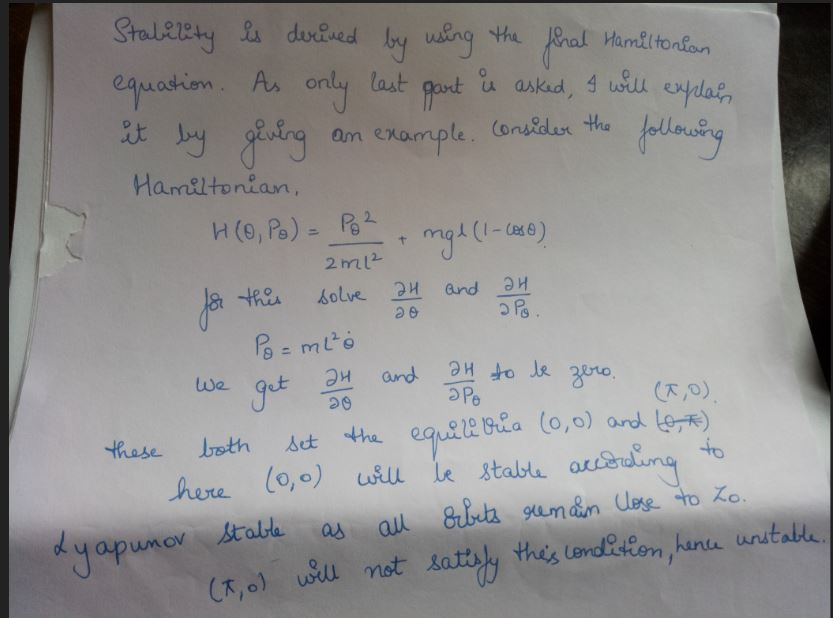# CAN YOU PLEASE JUST GIVE ME THE ANSWER FOR PART ( d) 2. Consider a bead...

CAN YOU PLEASE JUST GIVE ME THE ANSWER FOR PART ( d)2. Consider a bead of mass m sliding without friction on a wire that is bent into the shape of a parabola and spun with constant angular velocity ω about its vertical axis. Use cylindrical polar coordinates and let the equation of the parabola be : -kp (a) Find the Lagrangian in terms of p as the generalized coordinate. (b) Find the Hamiltonian for the system. (c) Is the Hamiltonian conserved? (d) From the Hamiltonian identify any equilibrium positions and analyze their stability.

as the last part of the question is asked, i am giving an idea to solve it. please apply this to your hamitonian equation and get the result. if you are unable to do it, please text. i am solving it by giving an example because this question has to be solved by solving all the remaining subparts which is difficult and so i adpoted this method. hope you understood.#### Earn Coin

Coins can be redeemed for fabulous gifts.